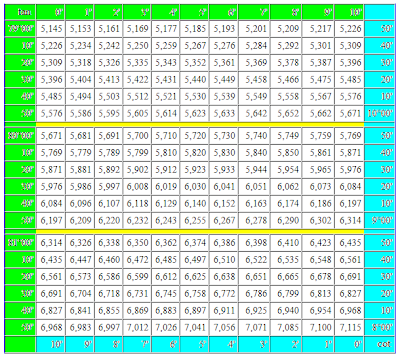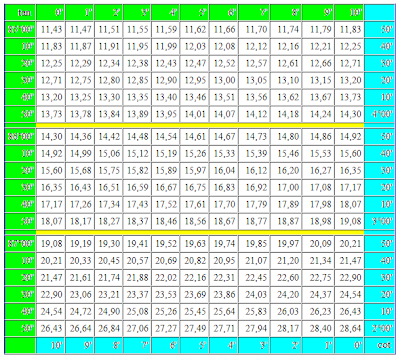## 2/04/2012

### Trigonometric table tangent cotangent in degrees

Trigonometric table tangent cotangent in degrees - it is the four-valued table of tangents and cotangents in degrees within one minute. A few examples are made at the beginning, as to use this table. For comfort in a table additional navigation elements are plugged with pointing by the name of the names of functions and values of minutes column-wise for every function. Value of degrees and minutes for every trigonometric function distinguished by different colors: for tangents - green, for cotangents - blue. Connotations for minutes are distinguished by yellow.

A trigonometric table for tangents and cotangents consists of two parts. The first part includes tangents from 0 to 75 degrees and cotangents from 15 to 90 degrees. Before to use this table, it is recommended on present examples to find the values of tangents and cotangents on a table and compare results. If all turned out correctly for you, means you can consider itself an experience user of table.At the use it is necessary to remember this table, that additional values one, two and three minutes for a tangent have a sign plus and at addition added and at deduction subtracted, for a cotangent they have a sign minus and at addition subtracted, and at deduction added. For verification compare the got result to the values of the same name trigonometric functions in the alongside located cells.

The second part of trigonometric table includes tangents from 75 to 90 degrees and cotangents from 0 to 15 degrees. To use this table some simpler, than by the first part.For a tangent 90 degrees and cotangent 0 degrees a value is not certain. It is so accepted to consider because task on dividing by a zero mathematicians until now so not succeeded to decide.

If you need a trigonometric table of sines and cosines, then she can be found on a separate page.